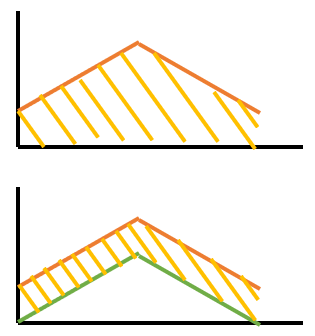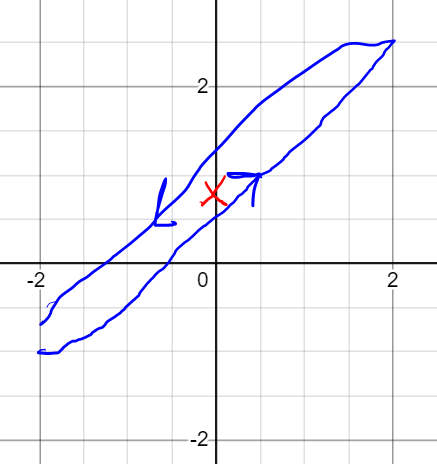# Some questions about encoder offset calibration algorithm and correct phase angle

Hi,
I studied the encoder offset calibration algorithm recently.

I know the calibration algorithm has 3 steps:

1. Align encoder angle `shadow_count_` to alpha axis.

2. Accumulate encoder angle and `num_steps` during turning rotor forward and backward.
i.e.
`encvaluesum += shadow_count_`
`num_steps++`

3. Calculate `phase_offset` and `phase_offset_residual`.

``````config_.phase_offset = encvaluesum / num_steps;
int32_t residual = encvaluesum - ((int64_t)config_.phase_offset * (int64_t)num_steps);
config_.phase_offset_float = (float)residual / (float)num_steps + 0.5f;  // add 0.5 to center-align state to phase
``````

then the electrical angle is corrected in 3 steps:

1. Calculate corrected encoder angle.
`int32_t corrected_enc = count_in_cpr_ - config_.phase_offset;`

2. Interpolate encoder angle.
`float interpolated_enc = corrected_enc + interpolation_;`
where `interpolation_` is 0.0, 0.5 or 1.0 is depended on moving.

3. Calculate electrical angle.
`float ph = elec_rad_per_enc * (interpolated_enc - config_.phase_offset_float);`

My questions are below:

1. Why just accumulate encoder angle and num_steps?
And could I treat the encoder angle aligned to the alpha axis as phase_offset?
Or accumulate the difference between the encoder angle and electrical angle * pole pairs then take average.

2. Why calculate phase_offset_float?
And could I ignore it?
Because I don’t think the residual divided by steps is a large number.

3. Why add 0.5 to phase_offset_float?
I can’t understand the meaning of `add 0.5 to center-align state to phase`.
Is the 0.5 value for interpolating?

4. Why interpolate the encoder angle?

There are many questions.
I will vary much appreciate if someone reply me.

In general you need to scan backwards and forwards to average out friction and other irregularities in the motor, locking in to a single point, say along alpha, is sometimes not so accurate (depending on motor).

When using hall sensors the space between states is large.

It’s just from how the state alignments are defined, what counts as “0” electrical angle.

Why interpolate the encoder angle?

Hall sensors are coarse.

Thanks, your explanations are very clear.
The friction and the hall sensor (low resolution) should be considered.

But, I didn’t understand why the offset is the average of the mechanical angle, not the average of the difference of the mechanical angle between the Electrical angle / pole pairs.where
the red line is the mechanical angle
the green line is the Electrical angle / pole pairs

In your plot you put x axis is time. But x axis is electrical angle. So the real plot looks like this:If you average the y coordinate of all the points in blue, you get the red X which is the offset we are looking for.

1 Like